# Yogurt

The board is 35 yogurts. How many boards of yogurts must be ordered for 886 people in the canteen?

Correct result:

x =  26

#### Solution:

$x= 886/35 = 25.314 \doteq 26$We would be pleased if you find an error in the word problem, spelling mistakes, or inaccuracies and send it to us. Thank you!Tips to related online calculators
Do you want to round the number?

## Next similar math problems:

• Into boxHow many cubes with an edge of 2.5 cm fit into a box measuring 11.6 cm; 8.9 cm and 13.75 cm?
• This year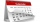This year 2020 is a leap year. How many leap years there had been be since 1904?
• Clay balls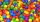How many clay balls with a radius of 1 cm can be made from a ball of clay with a radius of 8 cm?
• Hiking tripRosie went on a hiking trip. The first day she walked 18kilometers. Each day since she walked 90 percent of what she walked the day before. What is the total distance Rosie has traveled by the end of the 10th day? Round your final answer to the nearest ki
• RemainderWhat is the remainder of the division of natural numbers 293 and 7?
• Final exam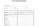At the final exam, the student answers from three areas, which are evaluated in a ratio of 1: 2: 2. What grade will John receive if he answered as follows: 3,1,2.
• AL wireWhat is the weight of an aluminum wire 250 m long with a diameter of 2 mm, if the density of aluminum is p = 2700 kg/m cubic. Determine to the nearest gram.
• My fatherMy father cut 78 slats on the fence. The shortest of them was 97 cm long, the longer one was 102 cm long. What was the total length of the slats in cm?
• Tiles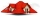The pupils of the building school have to calculate how many roof tiles they will need to cover the roof of the house in the shape of two rectangles measuring 10 x 7.2 m. One roof tile covers a rectangular area of 22 cmx32 cm. How many tiles will they nee
• The excavation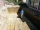The excavation for the city sewer was 38 m long, 2.2 m wide, and 3 m deep. a) How many cubic meters of soil were dredged b) How many journeys had to be made by one car when removing the soil, if they loaded an average of 4.5 m of cubic soil on each journe
• Growth of woodThe annual growth of wood in the forest is estimated at 2%. In how many years will make the forest volume double?
• NitrogenOne bag of urea containing 46 percent nitrogen weighs 25 kg. How many bags have to be purchased for fertilizing a field of 41003 square meters if the nitrogen dose is 50.0 kg per hectare?
• Pilsen circus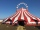The arrival of the circus in Pilsen was seen in the morning at 08:00 by a citizen of the city. He passed this information on 08:15 to three other residents of the city. Each of these three people then informed the other three residents at 08:30, and again
• Pilsen RegionBetween 2000 and 2001, 14 per mille of the population decreased in the Pilsen Region. In 2000, the Pilsen Region had 551281 inhabitants. If the declining trend continues the same (i. E. , 14 per mille of inhabitants per year), how many inhabitants will th
• Tiles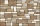The rectangular floor of the 6 x 1.8 m dimensions is to be covered with 50 cm square tiles. How many tiles will be needed?
• TableclothsThe restaurant has sixty-two square tablecloths with a side length of 150 cm and 36 rectangular tablecloths with dimensions of 140 cm and 160 cm. A) How many meters of hemming ribbon will be needed if we add 50 cm to each tablecloth? B) The ribbon sale in
• Paint cansHow many paint cans do we need to paint the floor in two rooms with dimensions of 6.8m x 4.5m and 6m x3.8m? One can arefor 6m².